## Sunday, October 28, 2012

### Review of FreeCalcFxc

Review: FreeCalcFxC

Platforms: iPhone and iPod Touch, will run on an iPad

Versions Available:
Free Version
\$0.99 Scientific Version
\$0.99 Financial Version

The only difference between the Free Version and the Scientific Version is that the Free Version is ad-supported.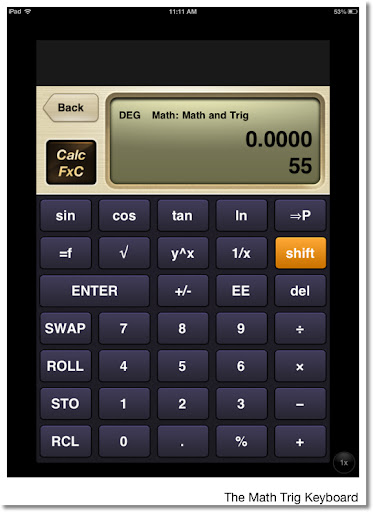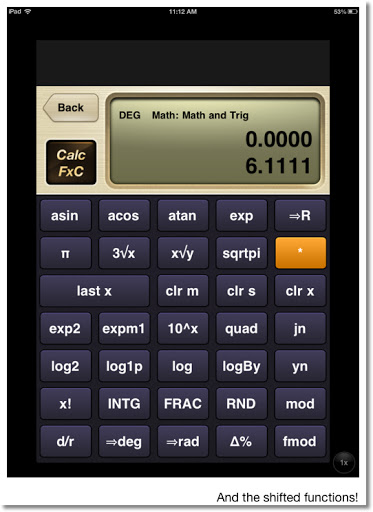Keyboard

The keyboard is a simple layout of 34 keys. The keys are labeled with one function. To access the shift function, press the gold shift key. The names of the shifted function replace the primary functions. Kudos for simplicity, however, my personal preference is to see both the primary and shift functions at the same time.

RPN and Algebraic Mode

The default mode is RPN (Reverse Polish Notation), but you can always enter an algebraic formula by pressing the "=f" key. The "=f" becomes the "=" to terminate entry of the formula.

Many, many, many calculators are available

Accessing the Focused Calculator list gives many calculators such as Math and Trig (the main calculator), Time Value of Money, Bonds, Statistics, Probability and Conversion calculators. For me the Conversion calculators leave a little to be desired, since there are no direct conversion keys attached to the default keyboard (luckily, that can be remedied!).

In addition to the Focused Calculators, CalcFxC gives formula template calculates. Among these formula calculators you have Percent Change, Distribution Functions, and the Quadratic and Cubic Equations.

The keyboard gives a good response when the "keys" are pressed. The screen is a two line (adjustable) screen which displays the y-stack and x-stack. The stack is four levels.

Help can be accessed by pressing and holding a key.

Real Numbers and Limits

The calculator operates on real numbers only. So, entering √-1 will return an error. As a consequence, the polynomial solvers return only real roots. The numbers range in the order of -10^-308 to 10^308.

There are no fractions or exact values of trig functions.

Math and Trig Keyboard

sqrtpi: takes the square root of the number then multiplies it by π
exmp1: e^x - 1, known on Hewlett Packard Calculators as the EXM1 function
log1p: ln(x + 1), known on Hewlett Packard Calculators as the LNP1 function
jn: Bessel Function of the First Kind, with x on the y-stack and the order n on the x-stack. jn also returns the Bessel Function of the Second Kind.
quad: Takes three arguments from the stack (a, b, c of ax^2+bx+c) and returns the real roots.

If you want to access the hyperbolic functions, you will need to call the Math and Hyperbolic calculator.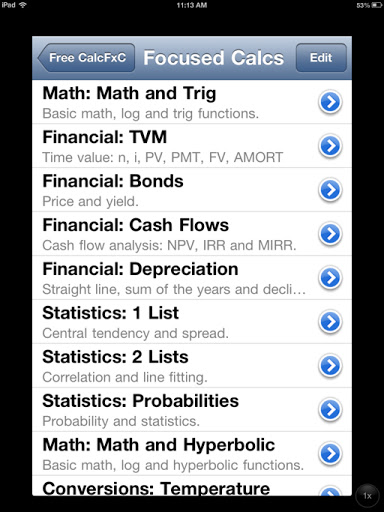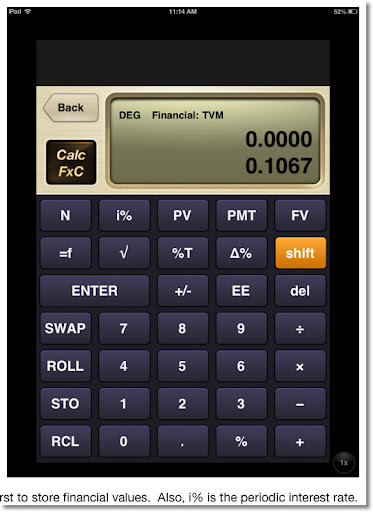Memory

The calculator has 27 memories: memories a through z, and a special register ra. Storage and recall arithmetic can be performed on register ra - no idea why (except for maybe programming limitations) CalcFxC decided to restrict this feature to one register.

Programmability in the Form of Customizable Calculators

CalcFxC does not offer "traditional" macro or program capability. Instead, CalcFxC offers the ability to edit and create custom keyboards. You can redefine the key's name and help screen, along with it's formula. You have access to all of the functions. To use the stack arguments, use rgx(), rgy(), rgz(), and rgt() for the x, y, z, and t stacks respectively. This is a fun feature for those who has ever wanted to design their own calculators, and I am one of them!

To emulate the stack operations properly, you will need to define the formulas for each stack.

For example, if I designate the comb function as the Combination function, I would use the following formulas:
x Formula: combin(rgy(),rgx())
y Formula: rgz()
z Formula: rgt()
t Formula: rgt()
Last x Formula: rgx()

Functions can be copied and pasted. You will need some experience with RPN calculators to take advantage of this feature.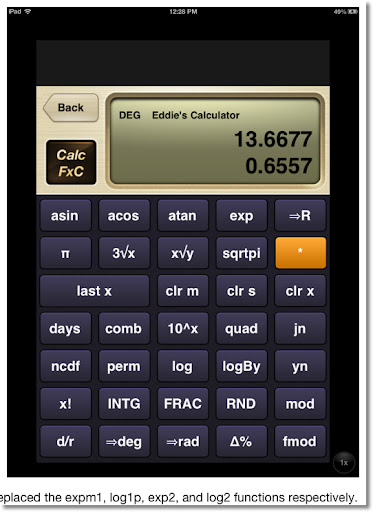Final Word

This app is enjoyable to use. I am probably going to spend an afternoon or evening making a custom calculator. RPN fans, this is a good calculator app to get.

Since I am not a fan of ads, I will pay the \$0.99 to get the non-ad version.

4.5 of 5 stars.

Eddie

This blog is property of Edward Shore. 2012.

## Wednesday, October 24, 2012

### Permutations: When Objects Repeat

Monday's blog entry (10/22/2012), Factorials and Arrangements of Unique Objects dealt with permutations of arranging a group of objects where all the objects are unique. Today's blog entry looks at three situations where objects can be repeated.

All the Choices are Available all the Time

This is where you make permutations in which all the objects are available for each slot. For example, let's take a five digit zip code. There are five slots and for each slot the 10 digits are available: 0, 1, 2, 3, 4, 5, 6, 7, 8, and 9.

For the first slot there are 10 choices. For each of the 10 choices, the second slot presents another 10 choices. For each of those 10 choices, the third slot presents another 10 choices. And so on.

The number of arrangements is: 10 × 10 × 10 × 10 × 10 = 100,000. There are 100,000 five-digit zip codes possible, 00000 to 99999.

Remember when calculating permutations, order matters. For our example, 11110, 11101, 11011, 10111, and 01111 are five different arrangements. The general rule is presented below.

Permutations where all the choices are available all the time = n^k

where
n = number of objects
k = number of slots

Objects Can Repeat, but with Some Restrictions

This is similar to the first situation in the way of calculating the number of permutations (arrangements).

Let's say we are on a very famous game show. One of it's mini games is played for a car valued anywhere from \$20,000 to \$59,999. We want to know how many possible prices for this game are possible.

Looking at the range from \$20,000 to \$59,999: we can see the choices for each of the five digits. The first digit must be a 2, 3, 4, or 5. The other four digits can be anything 0 through 9 (10 choices each).

The number of prices possible are 4 × 10 × 10 × 10 × 10 = 40,000.

So using a random guess, the contestant has a 1 in 40,000 choice in getting the price exactly right.

Another mini-game offers cars from \$11,111 to \$36,666 where the first digit is given to the contestant (1, 2, or 3) and the contestant tries to roll the other four digits using a single die. The die contains the numbers 1, 2, 3, 4, 5, and 6. Our task to find out how many prices are possible.

There are 3 choices for the first digit, and 6 choices for the other four digits.

The number of prices possible are 3 × 6 × 6 × 6 × 6 = 3,888.

This mini-game can be played up to 3,888 times before a price repeats.

Permutations where:
1) Different slots can have restrictions
2) Each choice is independent

P = (number of choices for slot 1) × (number of choices for slot 2) × ... × (number of choices for slot k)

Rearranging a Group of Objects where some of the Objects Repeat

In this situation we are finding the number of permutations of a group of objects, except some of the objects repeat.

Let's try to find the number of ways to arrange the letters in the word PHYSICS, removing any restriction that the arrangement has to make a sensible word (HYSSICP would count as arrangement).

In the word PHYSICS, there is 1 "P", 1 "H", 1 "Y", 2 "S"s, 1 "I", and 1 "C", for a total of 7 letters. PHYSICS counts as one permutation, regardless which "S" is used in each slot. We still have 7 letters, which can be arranged 7! = 5,040 ways, but have to account for the 2 "S"s.

The true number of ways to arrange the letters in the word PHYSICS is 2,520 ways.

7! / 2! = 5,040/2 = 2,520

Coincidentally, the calculation is really 7! / (1! × 1! × 1! × 2! × 1! × 1!). However, 1! = 1. Hence, 1! × 1! × 1! × 2! × 1! × 1! = 2!.

Let's take another example. Find the number of ways to arrange the letters in the "word" AAABB. In this example, there are 3 "A"s and 2 "B"s for a total of 5 letters.

If all the letters were unique, the number of ways is 5!. But we have to account for the repeats. Divide by 3! for the "A"s and 2! for the "B"s. The result is:

5! / (3! × 2!) = 120 / (6 × 2) = 10

There are only 10 ways to arrange the letters of the "word" AAABB. The ten are:

AAABB
AABAB
AABBA
ABABA
ABBAA

BABAA
BBAAA
ABBAA
AABBA
BAAAB

Finding the Number of Arrangements of Objects Where Some Objects Repeat

n! / ( (k_1)! × (k_2)! × ... × (k_m)! )

where:
n = the total number of objects, including repeated objects
m = the number of unique objects
k_1 = number of "k_1" objects
k_2 = number of "k_2" objects
and so on until...
k_m = number of "k_m" objects

The expression above is known as a multinomial coefficient.

I hope this day is well for each of you. Thank you for your comments and suggestions. Take care,

Eddie

Source:
Marcus, Daniel A. "Combinatorics: A Problem Orientated Approach" Mathematical Association of America, Washington DC. 1998.

This blog is property of Edward Shore, 2012.

## Tuesday, October 23, 2012

### Factorials and Arrangements of Unique Objects

Last weekend my dad asked me about my blog, and the last entry was about factorials of large numbers. Clicking on this link will take you there. On our way to classic car auto shop in Orange, CA; my dad and I talked about the factorials as I tried to come up with a way of finding applications using factorials. Last weekend became the inspiration for my blog entry. Love you, Dad.

Note: This blog entry will cover factorials of non-zero integers, that is n = 0, 1, 2, 3...

Factorials

The factorial of a non-negative integer, written with an exclamation mark after the number, is defined as:

n! = n × (n - 1) × (n - 2) × (n - 3) × ... × 3 × 2 × 1

Start by multiplying n by n less 1, then multiplying the product by n less 2, and repeat until you get to 1.

Examples
5! = 5 × 4 × 3 × 2 × 1 = 120
8! = 8 × 7 × 6 × 5 × 4 × 3 × 2 × 1 = 40,320

By definition, 0! = 1. Dr. James Tanton, Ph. D (www.jamestaton.com) gives an explanation why mathematicians choose to in video: Link to Video

Simply put, 0! = 1 is just defined this way.

Arrangements

In this section, we consider three common arrangement problems. The task is to find the number of possible permutations a group of objects can be arranged. A permutation is an arrangement where the order of which the objects are placed is important.

In this section, we are going to arrange all the objects.

Arrangement of Unique Objects

Consider a bookshelf that has room for five books. For simplicity, let's call the books A, B, C, D, and E (and not "Combinatorics", "The Irrationals", "Euclid's Number", "Programming", and "Making Soup for Dummies" like I originally planned.)

The for the first slot there are five choices. The second slot provides four choices. Whatever is available for the second slot depends on what book was put in the first slot. For example, if I choose to put book A in the first slot, the books B, C, D, and E are available for the second slot. Instead, if I choose to put book C in the first slot, then A, B, D, and E are available.

For each choice I make on the first slot, I get four choices for the second. Considering the first two slots alone, this gives me a total of 5 × 4 = 20 arrangements.

Continuing in this way, there are three choices for the third slot, two choices for the fourth slot, and whatever is left over gets the fifth slot.

So, the total number of arrangements of five books is:

5 × 4 × 3 × 2 × 1 = 5! = 120

Yes, 120 different arrangements. Since order is important, the arrangement is considered a permutation.

In general, working with n objects and n slots:
There are n objects for the first slot,
there are n - 1 objects for the second slot,
there are n - 2 objects for the third slot,
and so on,
until we reach 1 slot left for the last object.

Hence, the number of arrangements are:

n × (n - 1) × (n - 2) × ... × 1 = n!

Number of Permutations of n Unique Objects = n!

Arrangement of Unique Slots, Limited Spaces Available

Let's go back to our problem of arranging five books (A, B, C, D, and E) but this time, we only have three slots available.

For the first slot, I have 5 books available to choose from. Depending on what I choose, I will have 4 books for the second slot. Each of those 4 books present a choice of the 3 books for the last slot. Whatever is left either goes somewhere else in the house or gets donated.

The number of arrangements that are available to me has decreased due to the fact I only have three slots available. Hence, 5 × 4 × 3 = 60.

Observe if we multiply 5 × 4 × 3 by (2 × 1)/(2 × 1) we get:

5 × 4 × 3
= (5 × 4 × 3 × 2 × 1) / (2 × 1)
= 5! / (2 × 1)
= 5! / (5 - 3)!

Basically we are taking all of the ways 5 books can be arranged, and then dividing that number by all the ways the 2 books that are not going to be used can be arranged.

Doing the above in the general case is how we arrive at the formula for permutations:

nPk = n! / (n - k)!

where we have n objects and k slots to fill.

Permutations: nPk = n! / (n - k)! where n ≥ k. Order is important.

Until next time, have a great day!

Eddie

This blog is property of Edward Shore, 2012.

## Saturday, October 20, 2012

### Factorial of Large Numbers

Hi everyone! I am blogging from Coffee Klatch in San Dimas, CA! I was overdue for a latte. Their pumpkin brownies are to die for.

Factorial of Large Numbers

Calculators have an upper limit when working with numbers. Typically, the scientific calculators allow numbers up to 9.999999999 × 10^99. Anything over 10^100 is considered an overflow. Some of the higher graphing and CAS calculators allow for higher limits.

This means if you need to calculate anything over 69!, most calculators will give an "Overflow" error message.

Factorial:

The factorial of an integer n is the product:

n! = n × (n - 1) × (n - 2) × ... × 3 × 2 × 1

We can remedy this by using the laws of logarithms to calculate factorials of higher integers. Common logarithms (base 10) are used.

n! = n × (n - 1) × (n - 2) × ... × 2 × 1
log (n!) = log [ n × (n - 1) × (n - 2) × ... × 2 × 1 ]
log (n!) = log (n) + log (n - 1) + log (n - 2) + ... + log (2) + log (1)

log (n!) = ∑( log(k) from k = 2 to n)

Let T be this sum.

T = ∑(log (k) from k = 2 to n)

Note the following laws are used:

log (a × b) = log a + log b
log (1) = 0

If we take the anti-common logarithm of both sides, we get:

n! = 10^(∑( log(k) from k = 2 to n)
n! = 10^(T)
n! = 10^(frac(T) + int(T))
n! = 10^(frac(T)) × 10^(int(T))

Where:

(1) int(T) is the integer part of T. To find it, take the integer and ignore everything after the decimal point.

(2) frac(T) is the fractional part of T. To find this, take everything after the decimal point and ignore the integer part.

(3) int(T) + frac(T) = T

Example:
int(14.125) = 14
frac(14.125) = 0.125

A lot of programming and graphing scientific calculators have the int and frac functions. On most Hewlett Packard programming and scientific calculators, they are named IP and FP, respectively.

Example 1: 8!

Calculate 8! using the logarithm method.

T = ∑( log(k) for k = 2 to 8) = log 2 + log 3 + log 4 + log 5 + log 6 + log 7 + log 8 ≈ 4.605520523

In this case, int(T) = 4 and frac(T) = 0.605520523.

Then 8! ≈ 10^(0.65520523) × 10^4 ≈ 4.031999996 × 10^4

Because the accuracy of calculators and computer programs, I recommend that you round 10^(frac(T)) to a set amount of decimal places. I find that 5 decimal places works well. Then:

8! ≈ 4.03200 × 10^4

In fact, 8! = 40,320

Example 2: 111!

1. Calculate the sum ∑( log(k) from k=2 to 111). We find the answer to be 180.2462406.

2. Split the integer and fractional portions. Take the anti-common logarithm of the fractional portion. Hence, 10^0.2462406 ≈ 1.762952551. I round this answer to five decimal places to get 1.76295.

3. Answer: 111! ≈ 1.76295 × 10^180

In fact, using an HP 50g, 111! =
1,762,952,255,109,024,466,387,216,104,710,707,578,876,140,953,602,656,551,604,157,406,334,734,695,508,724,831,643,655,557,459,846,231,577,319,604,766,283,797,891,314,584,749,719,987,162,332,009,625,414,533,120,000,000,000,000,000,000,000,000

And yes, typing out this long number is not as easy it sounds.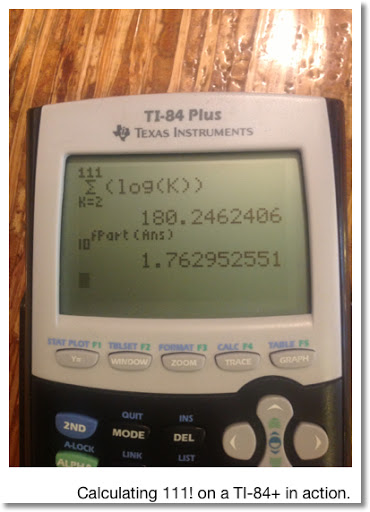Programming

Now we come to the programming portion of calculating n! using the logarithmic method.

This program was done on a HP 39gii, but can easily adapted on most graphing/programming calculators.

Program LFACT

EXPORT LFACT(N)
BEGIN
LOCAL T,M;
Σ(LOG(K)),K,2,N)→T;
ROUND(10^FRAC(T),5)→M;
MSGBOX(M + "10^" + INT(T));
RETURN {M, INT(T)};
END;

Here is a program in BASIC language. I used the App techBASIC from ByteWorks.
Everything after explanation points signify comments.

! Approximate Factorial (5 decimal mantissa plus exponent)
! Eddie Shore
! 10/20/2012

! To improve accuracy, set all variables as DOUBLE
01 DIM N as DOUBLE
02 DIM T as DOUBLE
03 DIM F as DOUBLE
04 DIM M as DOUBLE
05 DIM K as DOUBLE

10 PRINT "Factorial Program"
11 INPUT "N = "; N
12 T = 0

! Sum Loop
14 FOR K = 2 TO N
16 T = T + LOG10(K)
18 NEXT K

! Separation of T into its fractional and integer parts, we know T>0
20 M = INT(T)
22 F = T - M

! Round Fraction to five places - we have no ROUND function to work with
! 10^x when x<1 has a value less than 10
! Use string functions to round the answer to 5 decimal places (7 characters: the integer, decimal point, and five decimal places)
30 F = 10^F
32 F = VAL(LEFT(STR(F),7))

! Final results
40 PRINT "N! = "; F ; " x 10^"; M

Test Data:
8! = 4.0320 × 10^4
66! = 5.4434 × 10^92
122! = 9.87504 × 10^202
425! = 1.59775 × 10^934

Thank you as always, I appreciate the comments and compliments. Hope you enjoy the weekend.

Until next time,

Eddie

This blog is property of Edward Shore, 2012.

## Monday, October 15, 2012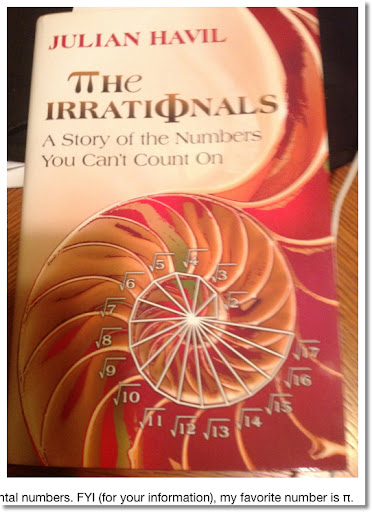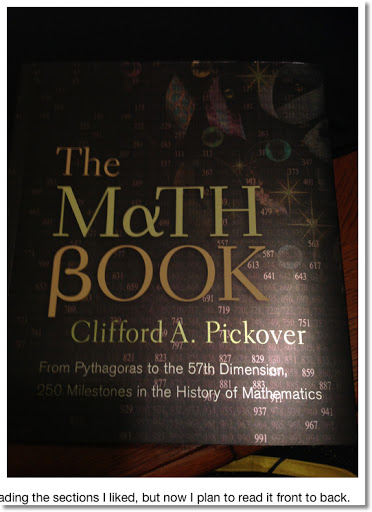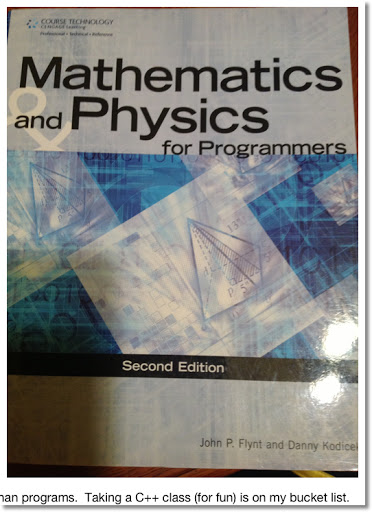Julian Havil. "The Irrationals: A Story of the Numbers You Can't Count On" Princeton University Press, 2012

Clifford A. Pickover "The Mαth βook". Sterling Publishing, 2009

John P. Flynt and Danny Kodicek "Mathematics and Physics for Programmers" 2nd Ed. Course Technology, 2012

Talk to you all soon, Eddie. Positive vibes and blessings.

## Thursday, October 11, 2012

### Calculator Tricks - Part 5

Welcome to Part 5 of Calculator Tricks

Part 5 will cover:
* Solving 2 by 2 equations
* Finding roots of the quadratic equations

The above tasks will be accomplished by using a simple calculator. That means we have only the arithmetic functions, square roots, percents, and memory to work with.

Solving 2 by 2 Equations

Suppose we are assigned the task solving for x and y:

Ax + By = E
Cx + Dy = F

This is a system of two simultaneous equations with two unknowns, x and y. We can use matrices to visualize this problem: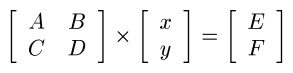Take the inverse of the coefficient matrix and left multiply both sides to get: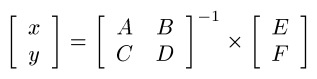We can find the inverse of the coefficient matrix by: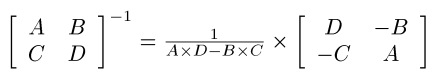The product A × D - B × C is called the determinant. Finally, we solve for x and y: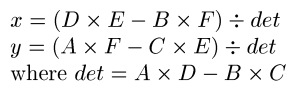Now that we have our general solutions, how do we tackle this on a simple calculator?

2 × 2 System of Equations

We only have one memory in the register to work with. Here is a strategy:

1. Calculate the determinant. Keystrokes: A × D - B × C =. Note this number down on paper. The calculator's memory will be required to find x and y.

2. Solve for x. Use the sequence:
MC
D × E = M+
B × F = M-
MR ÷ determinant =

3. Solve for y. Use the sequence:
MC
A × F = M+
C × E = M-
MR ÷ determinant =

---------------
Example: Solve the System

2x + 3y = -1
x - 3y = 2

We have A = 2, B = 3, C = 1, D = -3, E = -1, F = 2

Step 1:
Keystrokes:
MC
2 × 3 +/- = M+
3 × 1 = M-
MR

The determinant is -9. Write this result down.

Step 2:
Keystrokes:
MC
3 +/- × 1 +/- = M+
3 × 2 = M-
MR ÷ 9 +/- =

x ≈ 0.3333333

Step 3:
Keystrokes:
MC
2 × 2 = M+
1 × 1 +/- = M-
MR ÷ 9 +/- =

y ≈ -0.5555555

----------------

Let's tackle on how we find the roots of the equation:

Ax^2 + Bx + C = 0

Our focus for this blog entry will be finding real roots.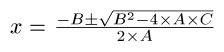We can derive our algorithm for finding both roots:

1. Calculate the discriminant. Store this result in the calculator's memory.

Keystrokes:
MC
B × = M+
4 × A × C = M-

2. Find the first root:

Keystrokes:
MR √ - B ÷ 2 ÷ A =

3. Find the second root:

Keystrokes:
MR √ +/- - B ÷ 2 ÷ A =
---------------
Example: Find the roots of

x^2 + 5x - 6 = 0

Let A = 1, B = 5, and C = -6

Step 1: Discriminant
MC
5 × = M+
4 × 1 × 6 +/- = M-

Discriminant = 49

Step 2: First Root
MR √ - 5 ÷ 2 ÷ 1 =

One of the roots is x = 1.

Step 3: Second Root
MR √ +/- - 5 ÷ 2 ÷ 1 =

The other root is x = -6

I hope this series is helpful. This series has showed how to make various calculations with a simple calculator: arithmetic procedures including fractions, area of a circle, distance between two points, calculating the total shopping bill, solving 2 x 2 simultaneous equations, and solving quadratic equations.

Until next time,

Eddie

This blog is property of Edward Shore, 2012.

### Calculator Tricks - Part 4

Welcome to Part 4 of Calculator Tricks. This is series is where accomplish mathematical tasks with a use of a regular, simple calculator. Part 4 will cover:

* Dealing with Percents
* Shopping
* Simple Interest

Dealing with Percents

With most calculators, if you have to add tax and subtract discounts, you can just execute the operation directly.

However, on some calculators, like the Casio SL-300VC, require a different sequence:
× n % + (to add) and × n % - (to subtract).

Here is how I prefer to work with percents, and it avoids the percent key altogether.

---------------

Let A be the number you want to add N% percent to it. In a shopping application, N represents the sales tax. In construction, N can be thought be allowance for waste.

A + N%
= A + (A × N/100)
= A × (1 + N/100)

Keystrokes: N ÷ 100 + 1 × A
---------------

Keystrokes: 10 ÷ 100 + 1 × 19.95

Result: 21.945

(On most calculators, 19.95 + 10 % works too)
---------------
Subtract Percent

Let A be the number you want to subtract N% from. In terms of shopping, N represents a discount.

A - N%
= A - (A × N/100)
= A × (1 - N/100)

Keystrokes: N ÷ 100 +/- + 1 × A
---------------
Example: Subtract 10% from 19.95.

Keystrokes: 10 ÷ 100 +/- + 1 × 19.95

Result: 17.955

(On most calculators, 19.95 - 10 % works too)
---------------

Shopping

Ever though what your bill would be as you shop? This section will show you what the potential bill will be and hopefully will lead you to make smart shopping decisions, and keep in budget.

Approach:
1. Clear Memory. We will use the memory register to keep track of our purchases.
2. Determine whether the item is subject to sales tax. You can press MR at any time to get a subtotal.
4. *If all of your items are subject to sales tax, add sales tax to the total.

If your purchases are "mixed", buying both non-taxable and taxable items (grocery store comes mind):

If the item or service is not subject to sales tax, use the keystroke sequence price M+ .

If the item or service is subject to sales tax, use the keystroke sequence tax factor × price M+ .

where tax factor = (1 + tax%/100)

In California, where I live, generally food, grocery items, and most services are generally not subject to sales tax, while sales of tangible goods are subject to sales tax.

Let's illustrate this strategy by a few examples.
---------------
Example 1: We are at a hardware store and purchasing the following items:

Hammer: \$19.99
Nails: \$3.95
Staple Gun: \$14.95
Measuring Tape: \$3.99

All items are subject to 8.5% sales tax.

Well, since we are dealing with just taxable purchases, we can total everything and add sales tax at the end.

Keystrokes:
MC
19.99 M+
3.95 M+
14.95 M+
3.99 M+
8.5 ÷ 100 + 1 = × MR =

Total: \$46.52
---------------
Example 2: From an office store, we are purchasing:

Ream of 500 sheets of paper: 3 at \$8.99 each
Pack of pencils: \$2.99
Pack of pens: \$3.95
Calculator: 2 at \$9.95 each

All items are subject to 8.5% sales tax. In this case, we can add the sales tax at the end.

Keystrokes:
MC
3 × 8.99 = M+
2.99 M+
3.95 M+
2 × 9.95 = M+
8.5 ÷ 100 + 1 = × MR =

Subtotal: \$53.81
With Sales Tax: \$58.38
---------------
Example 3: At a grocery store, we are purchasing:

Bag of Grapes: \$2.45
Apples: 5 at 99¢ each
Bananas: 6 at 24¢ each
Bread: \$4.09 with a 5% discount coupon
Water: 2 gallons at \$1.09 each
Box of Ziploc Bags: \$3.99 with a 50¢ coupon

Only the Ziploc bag is subject to 8.5% sales tax. Assume coupons take affect immediately (before sales tax). Here we must use the "mixed purchases" strategy. Enter \$3.49 for the Ziploc bags.

MC
2.45 M+
(grapes)
5 × 0.99 = M+ (apples)
6 × 0.24 = M+ (bananas)
5 ÷ 100 +/- + 1 = × 4.09 = M+ (bread)
2 × 1.09 = M+ (water)
8.5 ÷ 100 = + 1 = × 3.49 = M+ (Ziploc bags)
MR (final total)

Total bill: \$18.69
---------------

Simple Interest

The simple interest formula is:

I = P × R% × T

I = amount of interest
P = amount of the principal
R% = annual interest rate (as expressed as a decimal)
T = time, in years

The total amount paid is principal and interest, in other words, P + I.

---------------
Example 1: A bank makes a short term loan to Fred and Suzy of \$1,000. The bank charges 9.6% interest on short term loans. Fred and Suzy have to pay the loan in two months. If Fred and Suzy wait for the two months, how much interest have they paid?

We are looking for I. We have the following:
P = principal = 1000
R% = annual interest rate = 9.6/100
T = time = 2 months * 1 year/12 months

Note have to convert months to years. So the interest paid is:

Keystrokes:
1000 × 9.6 ÷ 100 × 2 ÷ 12 =

The total interest paid is \$16.
---------------
Example 2: Terrell is looking over his credit card bill. The balance is \$1,540.29. His credit card charges an annual rate of 15.99%. Terrell is planning to make a \$300.00 payment. Assuming Terrell does not use his credit card for the next month, what will be his balance?

Variables:
P = \$1,540.29 - \$300.00 = \$1,240.29
R% = 15.99%
T = 1/12 (1/12 of a year)

Keystrokes:
MC (Clear memory)
1540.29 - 300 = M+ (Subtract payment, interest will accrue on \$1,240.29.)
MR × 15.99 ÷ 100 ÷ 12 = (Months interest: \$16.53)
M+ MR (Add interest to memory)

Terrell's new balance next month would be \$1,256.82.
---------------
Example 3: Lita deposited \$500 in a Double Your Money CD. The bank will pay her \$1,000 when the CD doubles in value. The bank pays 7.5% interest on these deposits. How long will Lita wait?

This time we are looking for T.

Variables:
P = \$500
R% = 7.5%
I = \$500

Why is I = \$500? Lita deposits \$500 and will wait for her account to grow \$500, to earn \$500 in interest. Solving for T, time:

T = I / (P × R%/100) = I × (P × R%/100)^-1 = (P × R%/100)^-1 × I

Keystrokes:
500 × 7.5 ÷ 100 =
÷ ÷ = (37.5^-1)
× 500 = (Display: 13.333333)

So it takes 13 1/3 years to double Lita's CD. She may want to rethink this investment.
---------------

So this wraps up Part 4 of our Calculator Tricks series. Coming up in Part 5 we will tackle two common algebra problems.

Eddie

This blog is property of Edward Shore, 2012.

### Calculator Tricks - Part 3

Welcome to Part 3 of the Calculator Trick series using the Simple Calculator. This part covers:

* Squaring Numbers
* Reciprocals
* Geometric Applications

Just a reminder, a simple calculator has the following functions: arithmetic (+, -, ×, ÷), square root (√), percent (%), and memory (M+, M-, MR, MC). If your calculator has a MRC clear, this means press MRC once to recall memory, twice to clear it.

Squaring Numbers

---------------
To square a number, use the following key sequence:

× =
--------------
Example 1: 7^2

Keystrokes:
7 × =
---------------
Example 2: √(3^2 + 4^2) = 5

Use the memory keys to solve this problem. Squaring a number is multiplying a number by itself. Apply the square root last.

Keystrokes: MC (Again, always a good idea to clear memory to start)
3 × = M+ (3^2 = 9)
4 × = M+ (4^2 = 16)
MR (3^2 + 4^2 = 25)
(√25 = 5)
----------------

Reciprocals

---------------
To find the reciprocal of a number, use the following key sequence:

÷ ÷ =
(Yes, press the division key twice)
---------------
Example 1: 1/7 ≈ 0.142857

Keystrokes: 7 ÷ ÷ =
---------------
Example 2: 1/(1/5 + 1/3.2) ≈ 1.9512195

Strategy: Tackle the denominator first. Of course, anytime you have mixed operations, chances are you will be using the memory register.

Keystrokes:
MC
5 ÷ ÷ = M+ (1/5 = 0.2)
3.2 ÷ ÷ = M+ (adding 1/3.2 = 0.3125)
MR (1/5 + 1/3.2 = 0.5125 )
÷ ÷ = ( 1/(1.5 + 1/3.2) ≈ 1.9512195)
---------------

Geometric Applications

Area of the a Circle:
A = π r^2

where r is the radius and π is the constant pi. In this series, I am working with an 8 digit calculator, I will use the approximation π ≈ 3.1415927. I can use less digits, but I want as much accuracy as possible.

---------------
Area of a Circle: A = π r^2

Keystrokes: radius × = × 3.1415927 =
---------------
Example: Find an area of a circle with a radius of 14.5 inches.

Keystrokes: 14.5 × = × 3.1415927 =

The area is approximately 660.51986 square inches.
---------------

Distance between two Cartesian points (x1, y1) and (x2, y2):
√((x2 - x1)^2 + (y2 - y1)^2)

---------------
Distance between (x1, y1) and (x2, y2):

Keystrokes: MC
x2 - x1 = × = M+
y2 - y1 = × = M+
MR √

---------------
Example: Find the distance between the points (5,6) and (1,9).

Note: (x1, y1) = (5, 6) and (x2, y2) = (1, 9)

Keystrokes: MC
1 - 5 = × = M+
(Display: 16 M)
9 - 6 = × = M+ (Display: 9 M)
MR √ (Display: 5 M)

The distance between the points (5, 6) and (1, 9) is 5 units.
---------------

Right Triangles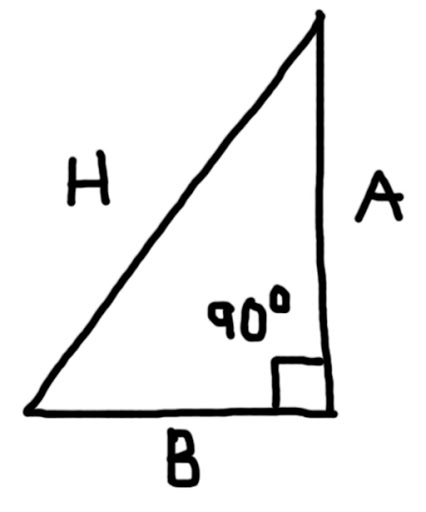Area = 1/2 × A × B

Pythagorean Theorem: H^2 = A^2 + B^2

---------------
With A = 3.9 inches, B = 2.4 inches. Find the area and the hypotenuse (H).

Area:
Keystrokes: 3.9 × 2.4 ÷ 2 =

The area is 4.68 square inches.

Finding the Hypotenuse:
Keystrokes: MC 3.9 × = M+
2.4 × = M+
MR √

The hypotenuse is approximately 4.5793012 inches.
---------------

Next time in Part 4, we will cover shopping and short-term loans with simple interest.

Eddie

This blog is property of Edward Shore, 2012.

### Calculator Tricks - Part 2

Fractions

Welcome to Part 2 of the Calculator Tricks series. We are using a simple ("four banger" calculator) to tackle common mathematical problems. If you missed Part 1,
you can check it right here by clicking on this link.

Simple Fractions

Simple calculators do not have the ability to display numbers as fractions, just their decimal equivalents.

Here are the decimal equivalent of some fractions that are handy to remember:

1/8 = 0.125
1/4 = 0.25
1/3 ≈ 0.3333333
3/8 = 0.375
1/2 = 0.5
5/8 = 0.625
2/3 ≈ 0.6666667
3/4 = 0.75
7/8 = 0.875

Remember the order you press the keys is critical, since the simple calculator operates in Chain Mode.

This blog assumes that you are working with an 8-digit calculator.

Adding and subtracting fractions will require the use of memory. Remember to always clear memory before beginning a calculation.

---------------

Keystrokes:
MC
(clear memory because we will need it)
A ÷ B = M+
C ÷ D = M+
MR

---------------
Subtracting Fractions: A/B - C/D

Keystrokes:
MC
A ÷ B = M+
C ÷ D = M-
MR

---------------
Example 1: 4/11 - 3/99 = 1/3 ≈ 0.3333333

The decimal equivalent (0.3333333 on a 8-digit calculator) is what we are after in this example.

Keystrokes:
MC
(Yes, I can't emphasize clearing the memory at the start enough!)
4 ÷ 11 = M+
3 ÷ 99 = M-
MR (Display: 0.3333333 M)
--------------
Example 2: 1/7 + 3/8 - 4/9 = 37/504 ≈ 0.073412698

We can use this technique to add and subtract more than two fractions.

Keystrokes:
MC
1 ÷ 7 = M+
3 ÷ 8 = M+ (adding 0.375)
4 ÷ 9 = M- (subtracting 0.4444444)
MR (Display: 0.0734127 M)

Depending on the calculator the last digit may be rounded or not. This result is correct to eight decimal places.

2. Multiplying and Dividing Fractions

Let's multiply the fractions A/B × C/D. Using some algebra, we can simplify this expression.

A/B × C/D
= (A × C)/(B × D)
= (A × C)/B × 1/D
= (A × C) ÷ B ÷ D

---------------
Multiplying Fractions: A/B × C/D

Keystrokes: A × C ÷ B ÷ D =

Multiply the numerators, divide the denominators.
---------------
Example: 4/7 × 2/3 = 8/21 ≈ 0.3809524

Keystrokes: 4 × 2 ÷ 7 ÷ 3 =
---------------

Let's divide the fraction A/B by C/D. (Calculate A/B ÷ C/D) To divide number by a fraction, multiply it by the fraction's reciprocal.

A/B ÷ C/D
= A/B × D/C
= (A × D)/(B × C)
= (A × D)/B × 1/C
= (A × D) ÷ B ÷ C

----------------
Dividing Fractions: A/B ÷ C/D

Keystrokes: A × D ÷ B ÷ C =
---------------
Example: 7/9 ÷ 2/5 = 7/9 × 5/2 = 35/18 ≈ 1.9444444

Keystrokes: 7 × 5 ÷ 9 ÷ 2 =
----------------

Mixed Fractions

A strategy is to to covert mixed fractions to simple (improper fractions) first.

For the mixed fraction A B/C is converted into the simple fraction (A × C + B)/C. Adding two mixed fractions will require the use of the memory register.

---------------

A B/C + D E/F = (A × C + B)/C + (D × F + E)/F

Keystrokes: MC
A × C + B ÷ C = M+
D × F + E ÷ F = M+
MR

---------------
Example: 8 1/9 + 7 3/5 = 15 32/45 ≈ 15.711111

Keystrokes: MC
8 × 9 + 1 ÷ 9 = M+
7 × 5 + 3 ÷ 5 = M+
MR

---------------

Multiplying Mixed Fractions

Unlike multiplying simple fractions, multiplying mixed fractions will require the use of memory. We can get a form for an algorithm by simplifying.

A B/C × D E/F
= (A × C + B)/C × (D × F + E)/F
= ((A × C + B) × (D × F + E))/(B × C)
= ((A × C + B) × (D × F + E)) ÷ B ÷ C

---------------
Multiplying Mixed Fractions

A B/C × D E/F = ((A × C + B) × (D × F + E)) ÷ C ÷ F

Keystrokes:
MC
A × C + B M+
D × F + E =
× MR =
÷ B ÷ F

---------------
Example 1: 4 1/2 × 8 1/3 = ((4 × 2 + 1) × (8 × 3 + 1)) ÷ 2 ÷ 3 = 37.5

Keystrokes:
4 × 2 + 1 = M+ (Store 4 × 1 + 2 = 9 in memory)
8 × 3 + 1 = (Display: 25 M)
× MR = (numerator is done, Display: 225)
÷ 2 ÷ 3 = (no divide by the denominators)

The display should be 37.5 (with the memory indicator). This is a complex algorithm, so it will take some practice. Let's do another example.
---------------
Example 2: 5 5/9 × 16 2/3 = ((5 × 9 + 5) × (16 × 3 + 2)) ÷ 9 ÷ 3 ≈ 92.59259

Keystrokes:
5 × 9 + 5 = M+ (Store 4 × 1 + 2 = 50 in memory)
16 × 3 + 2 = (Display: 50 M)
× MR = (numerator is done, Display: 2500 M)
÷ 9 ÷ 3 = (no divide by the denominators)

The display should have 92.59259.
---------------

In the next example we will add and multiply mixed fractions. Be willing to write immediate results on a note pad with complex problems.

---------------
Example 3:
4 1/8 + 3 3/7 × 6 2/3 = 1511/56 ≈ 26.982143

The Order of Operations says we must multiply the mixed fractions first. However, we only have one memory register, and we don't have the ability to "switch" whatever is in the display with memory. Tackling this problem requires a plan. Here is one way:

1. Change 4 1/8 into a fraction and write it down.
2. Multiply the mixed fractions 3 3/7 × 6 2/3.
3. Add the resulting decimal equivalent of 4 1/8 to the result obtained from step 2.

Step 1 Keystrokes:
4 × 8 + 1 ÷ 8 =
Note the result, which is 4.125. Don't store this number in memory, but on a notepad.

Step 2 Keystrokes:
MC
3 × 7 + 3 = M+
(Display: 24 M)
6 × 3 + 2 = (Display: 20 M)
× MR = (Display: 480 M)
÷ 7 ÷ 3 = (Display: 22.857142 M)

Step 3 Keystrokes:
Add 4.125 obtained from step 1 to 22.857142 obtained from step 2.
+ 4.125 = (Display: 26.982142 M)

We have arrived at our result.

The lesson here is to plan your calculation.

Another strategy to calculate 4 1/8 + 3 3/7 × 6 2/3 is to change all fractions to their decimal equivalents first, noting the decimal equivalents on paper. Let's see how this works out:

1. Change each mixed fraction to their decimal equivalent.
2. Complete the calculation.

Step 1 Keystrokes:
4 × 8 + 1 ÷ 8 = (4 1/8 = 4.125)
3 × 7 + 3 ÷ 7 = (3 3/7 ≈ 3.4285714)
6 × 3 + 2 ÷ 3 = (6 2/3 ≈ 6.6666666)

Now we have 4.125 + 3.4285714 × 6.6666666

Step 2 Keystrokes:
3.4285714 × 6.6666666 + 4.125 =
Result: 26.982142

The next time we meet, we will work with square numbers, reciprocals, and some geometry.

Eddie

This blog is property of Edward Shore, 2012.

### Calculator Tricks - Part 1

First of all, I am home taking care of my dad today. He's doing fine, recovering from surgery.

I also want to thank everyone who reads this blog and leaves comments. I appreciate the input and conversation.

Eddie

---------------

Introduction

Don't have a scientific calculator and only have a simple calculator with you? Interested in maximizing the abilities of a simple calculator? Want to impress your friends and co-workers? This series is for you.

What do I mean by a simple calculator? it is the calculator that you find everywhere, not just produced by the big calculator manufacturers Hewlett Packard, Texas Instruments, Sharp, and Casio, but as a novelty item from companies with almost any color or design you like. If you prefer, there are thousands of calculator apps on almost any cell phone, tablet, or iPod Touch. I refer to this type of calculator as a "four-banger" because it's primary functions are the arithmetic functions (+, -, ×, ÷).

Part 1 will cover:

* Chain Mode
* The Memory Keys
* Arithmetic Operations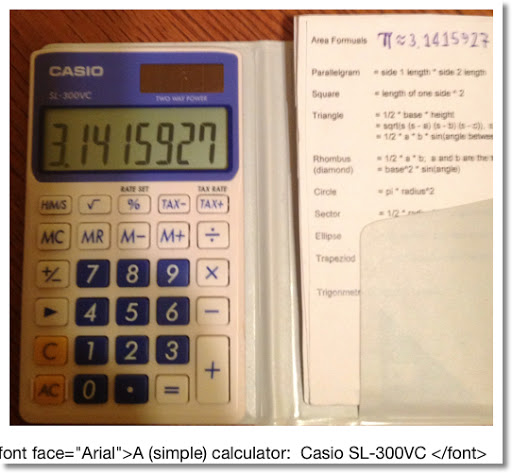Chain Mode

I estimate that 99% of simple calculators operate on chain mode. That is calculations take place as you enter them, without regard to the Order of Operations. You may remember the expression "My Dear Aunt Sally" or it's expanded version "Please Excuse My Dear Aunt Sally" as a mnemonic for the Order of Operations.

Order of Operations:
Please (everything in Parenthesis gets priority)
Excuse (Exponents, roots, [sine, cosine, tangent, logarithm])
My Dear (Multiplication, Division)

Here is how to know whether your calculator is operating in Chain Mode:

Type, in order: 4 + 2 × 3 =

Now using the proper order of operations, 4 + 2 × 3 = 10. However, calculators in chain mode don't "know" the order of operations. In that case, that calculator completes 4 + 2 first before multiplying the result by 3, giving a result of 18.

So if you type 4 + 2 × 3 = , in that order, and get 18, your calculator operates in Chain Mode. This means we have to manually take the Order of Operations into account to ensure we get the correct answer of 10.

One way to do this is rearrange the expression to 2 × 3 + 4. Typing the expression in this order will give us the correct answer, 10.

For this series, we will work with calculators operating in Chain Mode, which covers about 95% of simple calculators.

The Memory Keys

The simple calculator has four memory operations:
M+: Add whatever is in the display to Memory.
M-: Subtract whatever is in the display from Memory.
MR: Recall the contents of Memory.
MC: Clears the contents of Memory.

Often, you will see the key MRC. Press this key once to recall the contents of memory, twice to clear it.

For this series, I will keep MR and MC separate. Just remember if you are working with the MRC key, pressing MRC twice will clear memory.

The memory register is a key to advanced calculations on a simple calculator. One, it can help keep our calculations in proper operation. Second, it can store a number for later use.

Your calculator will give an indicator ("M" or "MEMORY") whenever a number other than zero is stored in memory. The memory is consider cleared when 0 is stored and the calculator does not display a memory indicator.

On to the Arithmetic Section.

Arithmetic Section

Let's tackle some common arithmetic calculations with the simple calculator to get the correct answers. Remember the order you press the keys is critical, since the simple calculator operates in Chain Mode.

For each section, I will give a proper keystroke to tackle the problem. Then I will give an example. Each capital letter (A, B, C, etc.) represents a variable.

1. A × B + C

This is a fairly simple expression. Just keep "Please Excuse My Dear Aunt Sally" in mind and you're gold.

---------------
A × B + C

Keystrokes: A × B + C =
---------------
Example: 8 × 6 + 3 = 51

Keystrokes: 8 × 6 + 3 =

The result is 51, which the correct result with the Order of Operations.

2. A × B + C × D

Now we have two multiplications to do before the addition. This is where the memory keys (M+, M-, MR, MC) come in handy. Before you start any operation involving memory, clear it first! Remember if your calculator has a MRC key, press it twice to clear memory.

---------------
A × B + C × D

Keystrokes:
MC
A × B = M+
C × D = M+
MR

---------------
Example: 4 × 1.95 + 3 × 0.99 = 10.77

Key:
MC
4 × 1.95 = M+
(Display: 7.8 M)
3 × .99 = M+ (Display: 2.97 M)
MR (Display: 10.77 M)

3. A × B - C × D

This is similar to problem 2.

---------------
A × B - C × D

Keystrokes:
MC
A × B = M+
C × D = M-
MR

---------------
Example: 4 × 8.25 - 3 × 1.95 = 27.15

Key:
MC (always want to clear memory before beginning)
4 × 8.25 = M+ (Display: 33 M)
3 × 1.95 = M- (Display: 5.85 M)
MR (Display: 27.15 M)

4. A × (B + C)

Note the parenthesis around the addition of B and C. This time we work the addition first.

---------------
A × (B + C)

Keystrokes: B + C × A
---------------
Example: 8 × (6 + 3) = 72

Keystrokes: 6 + 3 (Display: 9)
× 8 = (Display: 72)

5. (A + B) ÷ (C + D)

A strategy is to work the denominator first, store the result in memory. Then work left to right.

---------------
(A + B) ÷ (C + D)

Keystrokes: MC
C + D = M+
(store denominator in memory)
A + B
÷ MR =

---------------
Example: (48 - 16) ÷ (3 + 1) = 8

Keystrokes: MC
3 + 1 = M+
(4 is stored in memory)
48 - 16 (working the numerator)
÷ MR = (divide by memory)

Display: 8 M

This ends Part 1 of our series. In part 2, we will work with fractions. Thanks,

Eddie

This blog is property of Edward Shore, 2012.

## Tuesday, October 2, 2012

### Distribution of Digits for π, e, Euler's Constant, and √2

Here are four famous numerical constants, extended to 50 decimal places: π, e, γ, and √2. For each constant, the approximation will be listed and a histogram of each of the 50 decimal places and the integer part (51 digits in total) will be presented.

Pi
π ≈ 3.14159 26535 89793 23846 26433 83279 50288 41971 69399 37510

Digit Distribution: (digit: number of occurrences)
0: 2
1: 5
2: 5
3: 9
4: 4
5: 5
6: 4
7: 4
8: 5
9: 8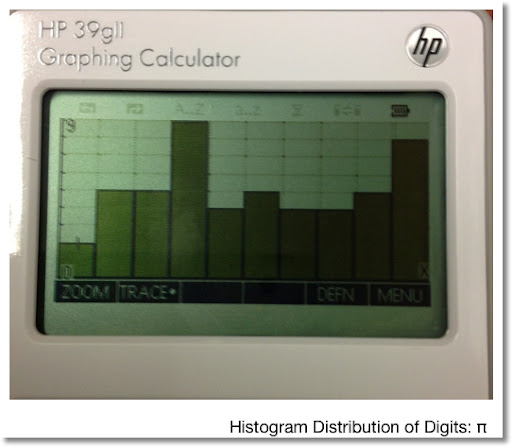e

e ≈ 2.71828 18284 59045 23536 02874 71352 66249 77572 47093 69995

Digit Distribution:
0: 3
1: 3
2: 8
3: 4
4: 5
5: 6
6: 4
7: 7
8: 5
9: 6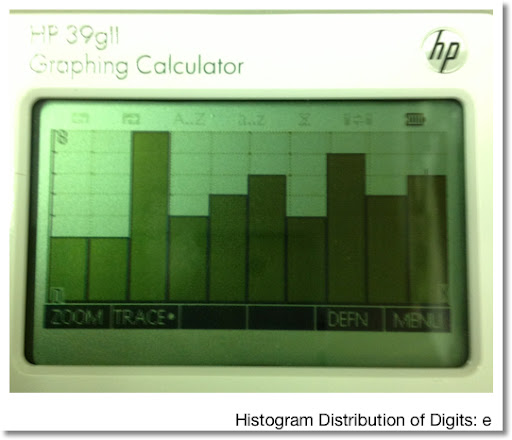γ (Euler's Constant)

γ ≈ 0.57721 56649 01532 86060 65120 90082 40243 10421 59335 93992

Digit Distribution:
0: 9
1: 5
2: 7
3: 5
4: 4
5: 6
6: 5
7: 2
8: 2
9: 6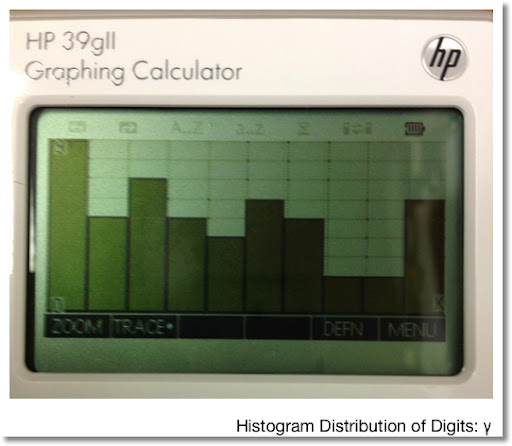√2

√2 ≈ 1.41421 35623 73095 04880 16887 24209 69807 85696 71875 37694

Digit Distribution:
0: 5
1: 5
2: 4
3: 4
4: 5
5: 4
6: 6
7: 6
8: 7
9: 5

Of the four constants presented, √2 has the most even distribution, at far as the first 50 decimal points are concerned.

Fun Fact: 99/70 gives √2 accurate to four decimal places.
99/70 = 1.41428 571428...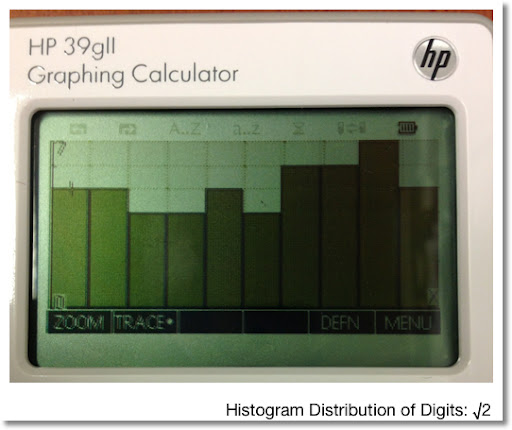Resources

π, e, γ

Zwillinger, Daniel. CRC - Standard Mathematical Tables and Formulae 32nd Edition. CRC Press, Boca Raton, FL. 2012

√2

√2 Wikipedia: Retrieved October 2, 2012.

Enjoy! This is something I wanted to do for a while, as I am fascinated by numerical constants.

Eddie

This blog is property of Edward Shore, 2012.

### HP 15C: Error Function and Lower Tail Normal Cumulative Function

HP 15C:   Error Function and Lower Tail Normal Cumulative Function Formulas Used  Error Function  erf(x) = 2 ÷ √π * ∫( e^(-t^2) dt, t = 0 t...